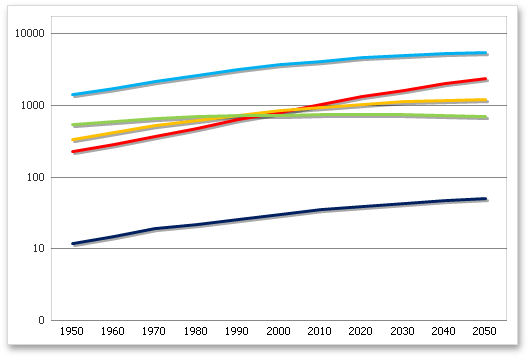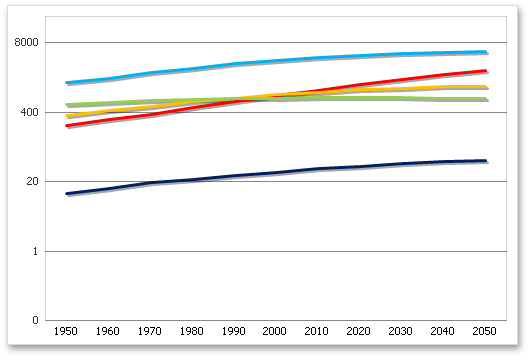.NET Framework 4.5.2+

# AxisScaling.LogBase Property

Gets or sets a value specifying a logarithmic base for the logarithmic axis.

## Declaration

``double LogBase { get; set; }``

## Property Value

Type Description
Double

A Double value that is the logarithmic base. This value must be greater than or equal to two.

## Remarks

If the AxisScaling.LogScale property is set to true, use the LogBase property to specify the logarithmic base.

With the LogBase property, you can define a base value as a logarithm that will be used to calculate the axis values.

The images in the table below demonstrate how the LogBase property works.

LogBase = 10 LogBase = 20## Example

The example below demonstrates how to create a simple line chart and change the value axis to logarithmic using the AxisScaling.LogScale property. The logarithmic base, defined by the `AxisScaling.LogBase` property, is set to 10.

``````Dim worksheet As Worksheet = workbook.Worksheets("chartTask5")
workbook.Worksheets.ActiveWorksheet = worksheet

' Create a chart and specify its location.
Dim chart As Chart = worksheet.Charts.Add(ChartType.Line, worksheet("B2:D8"))
chart.TopLeftCell = worksheet.Cells("F2")
chart.BottomRightCell = worksheet.Cells("L15")

' Set the logarithmic(Log10) type of scale.
chart.PrimaryAxes(1).Scaling.LogScale = True
chart.PrimaryAxes(1).Scaling.LogBase = 10

' Set the position of the legend on the chart.
chart.Legend.Position = LegendPosition.Bottom
``````

The following code snippets (auto-collected from DevExpress Examples) contain references to the LogBase property.

Note

The algorithm used to collect these code examples remains a work in progress. Accordingly, the links and snippets below may produce inaccurate results. If you encounter an issue with code examples below, please use the feedback form on this page to report the issue.